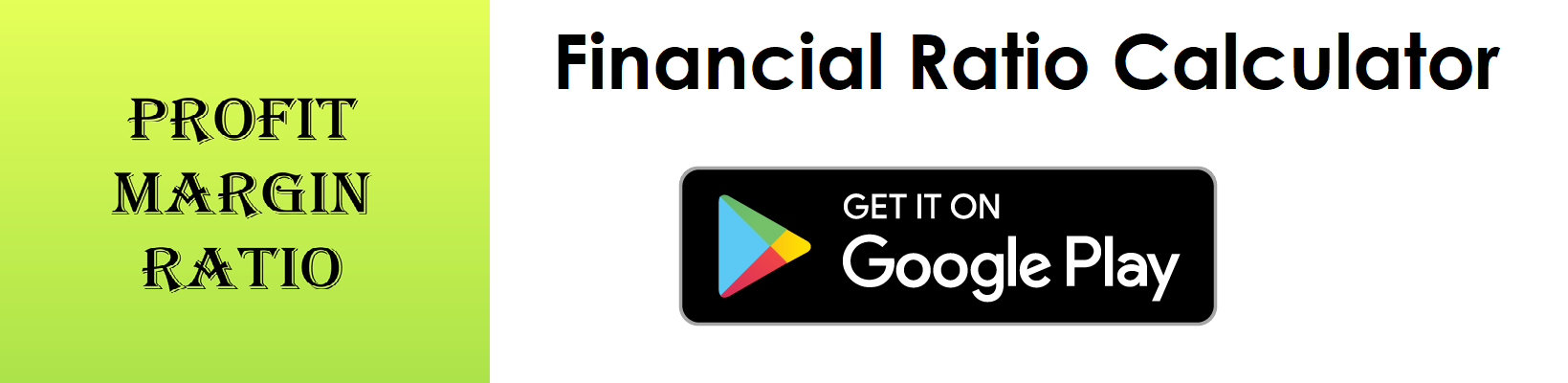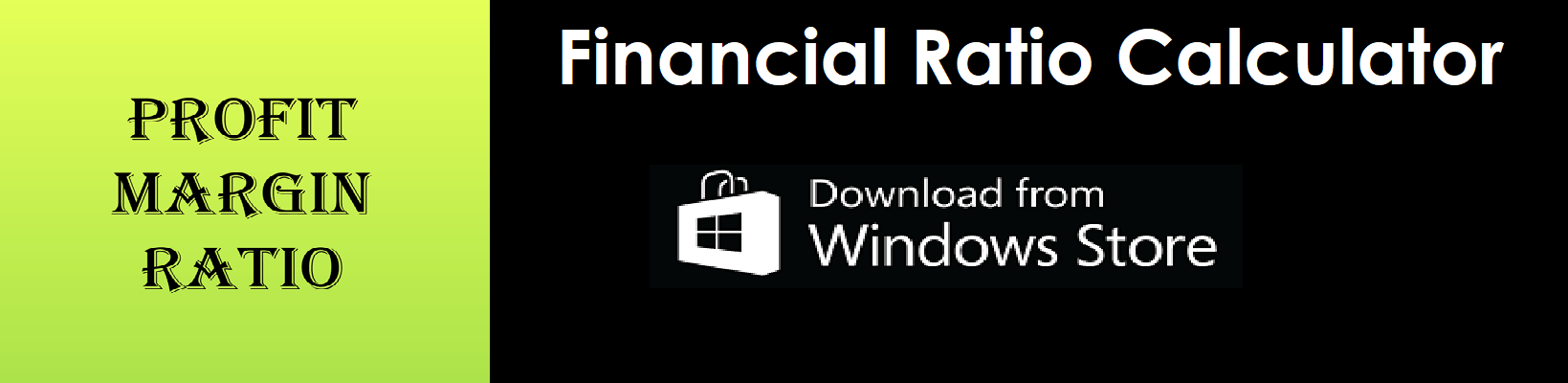# Quick Ratio Calculator

Key in the Current Assets, Inventory and Current Liabilities Values from your Balance Sheet to the respective fields given below and then click Calculate to get the desired result.

##### Quick Ratio Calculator - Glossary:
Using our quick ratio calculator, helps to compare, measure, understand the overall health of the business.

##### Quick Ratio:
Shows the proportion of quick assets to current liabilities. In simple words, it is a measure to check how well a company can quickly convert its asset into cash to pay off its current liabilities.

##### How to calculate?
Formula:
Quick Ratio = Quick Assets/Current Liabilities
This is a balance sheet component; the values are commonly stated against current assets, current liabilities and total assets.
Note:
Quick Assets = total current assets value - total inventory and any current prepaid assets value.

##### Quick Assets:
Quick assets are current assets that can be converted to cash in short-term. Simply subtract inventory and any current prepaid assets from the current asset total.

##### Current Assets:
This is a balance sheet component - cash, cash equivalents, account receivables, merchandise inventory and marketable securities can easily be converted into cash in the short term.

##### Inventory:
Inventory is the finished goods that can be sold immediately.

##### Current Liabilities:
This is a balance sheet component - accounts payable, tax payable (sales, payroll and other taxes) that are due in short-term.

##### Example:
Quick ratio for a company with a total current assets of \$240,000, merchandise inventory of \$40,000 and current liabilities of \$105,000 is 1.9 : 1. It means \$1.9 of quick assets available to cover each \$1 of short-term debt (current liabilities).

# Download our Mobile Apps - today.